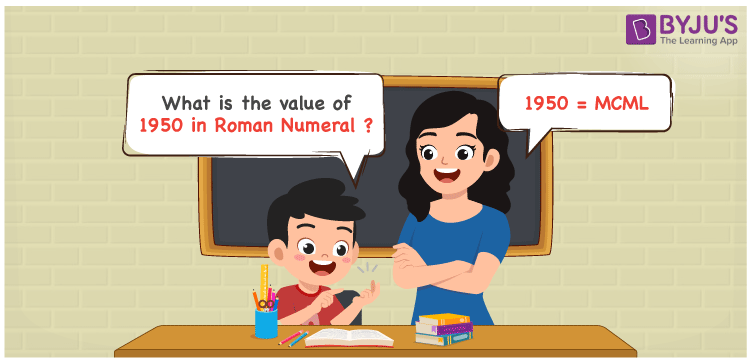# 1950 in Roman Numerals

1950 in Roman numerals is MCML. To convert 1950 to Roman Numerals, write it in expanded form, i.e. 1950 =1000 + 900 + 50, then replace the changed integers with corresponding roman numerals to get 1950 = M + CM + L = MCML. In this post, we’ll show you how to convert 1950 to Roman numerals accurately..

 Number Roman Numeral 1950 MCML

## How to Write 1950 in Roman Numerals?In order to write 1950 in Roman Numerals, it has to be written in expanded form, i.e.

1950 = 1000 + 900 + 50

1950 = M + CM + L

1950 = MCML

## Video Lesson on Roman Numerals